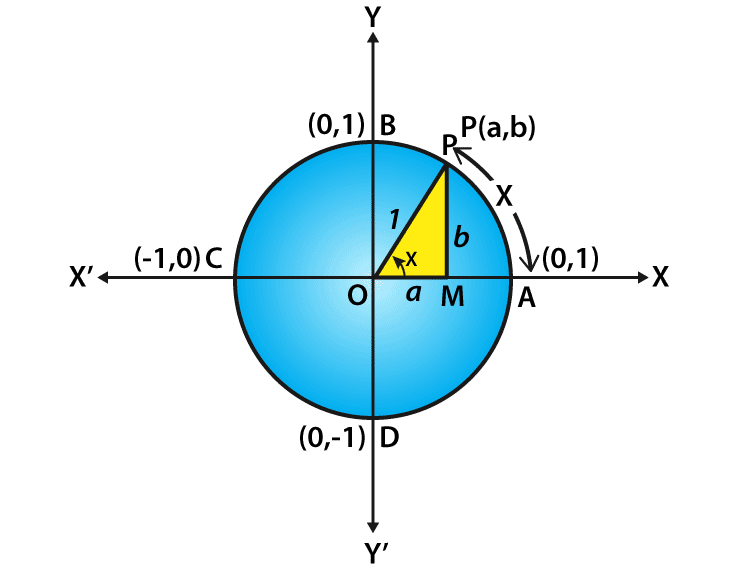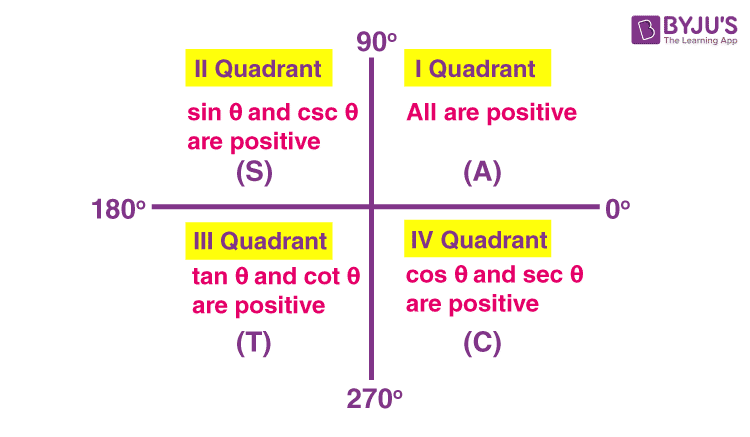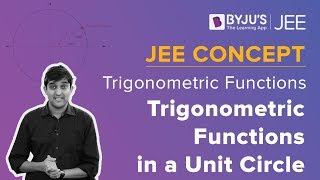# Sign of Trigonometric Functions

In trigonometry, there are six functions, namely sin, cos, tan, cosec, sec and tan. We can determine the sign of the trigonometric function with the help of a unit circle. Let P(a, b) be a point on the unit circle with the centre at the origin as shown in the figure below:For every point P (a, b) on the unit circle, – 1 ≤ a ≤ 1 and – 1 ≤ b ≤ 1, we have – 1 ≤ cos x ≤ 1 and –1 ≤ sin x ≤ 1 for all x. Also, we can define the values of a and b in different quadrants as:

First quadrant: 0 < x < π/2 ⇒ a and b are positive

Second quadrant: π/2 < x < π ⇒ a is negative and b is positive

Third quadrant: π < x < 3π/2 ⇒ a and b are negative

Fourth quadrant: 3π/2 < x < 2π ⇒ a is positive and b is negative

## Sign of Trigonometric Functions in Different Quadrants

The sign of trigonometric functions in each quadrant are given in the figure below.In the first quadrant all functions are positive, in the 2nd quadrant only sin and cosec are positive, in the 3rd quadrant only tan and cot are positive, whereas in the 4th quadrant only cos and sec are positive. Thus, we can tabulate the signs of different trigonometric functions in different quadrants as follows:

 Quadrant I Quadrant II Quadrant III Quadrant IV sin θ +ve +ve -ve -ve cos θ +ve -ve -ve +ve tan θ +ve -ve +ve -ve cosec θ +ve +ve -ve -ve sec θ +ve -ve -ve +ve cot θ +ve -ve +ve -ve

Go through the example given below to understand how to determine the sign of different trigonometric functions in different quadrants.

Example:

If cot x = -5/12, x lies in the fourth quadrant, then find the value of cosec x.

Solution:

Given,

cot x = -5/12

We know that,

cosec2x – cot2x = 1

cosec2x = 1 + cot2x

= 1 + (-5/12)2

= 1 + (25/144)

= (144 + 25)/144

= 169/144

cosec x = √(169/144) = ±13/12

Given that x lies in the fourth quadrant and cosec x is negative in the fourth quadrant.

Therefore, cosec x = -13/12

Similarly, we can solve various types of trigonometry problems quickly. These are useful in determining the values of trigonometry functions which are dependent on other functions and trigonometry angles also.

### Watch The Below Video To Understand Trigonometric Functions in Unit Circle” />

For more interesting concepts of trigonometry, download BYJU’S – The Learning App today!